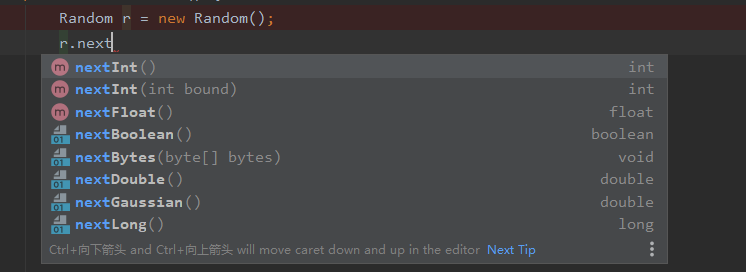• JAVA随机数生成方式一：java.lang.Math.Random方式二：java.util.Random JAVA中有两种方式生成随机数： 方式一：java.lang.Math.Random 调用Math.Random()函数能够返回带正号的double值，该值大于等于0.0且小于1.0，...
JAVA随机数生成方式一：java.lang.Math.Random方式二：java.util.Random
JAVA中有两种方式生成随机数：
方式一：java.lang.Math.Random
调用Math.Random()函数能够返回带正号的double值，该值大于等于0.0且小于1.0，即取值范围是[0.0,1.0)的左闭右开区间，返回值是一个伪随机选择的数，在该范围内（近似）均匀分布。例子如下：
package IO;
import java.util.Random;

public class TestRandom {

public static void main(String[] args) {
// 案例1
System.out.println("Math.random()=" + Math.random());// 结果是个double类型的值，区间为[0.0,1.0）
//例如：生成0-2的随机数
int num = (int) (Math.random() * 3); // 注意不要写成(int)Math.random()*3，这个结果为0，因为先执行了强制转换
System.out.println("num=" + num);


方式二：java.util.Random
Random()有两种构造方法：

Random()：创建一个新的随机数生成器。
Random(long seed)：使用单个 long 种子创建一个新的随机数生成器。

我们可以在构造Random对象的时候指定种子（这里指定种子有何作用，请接着往下看），如：Random r1 = new Random(20);
或者默认当前系统时间的毫秒数作为种子数:Random r1 = new Random();
需要说明的是：你在创建一个Random对象的时候可以给定任意一个合法的种子数，种子数只是随机算法的起源数字，和生成的随机数的区间没有任何关系。如下面的Java代码：
Random rand =new Random(25);
int i;
i=rand.nextInt(100);

初始化时25并没有起直接作用（注意：不是没有起作用）,rand.nextInt(100);中的100是随机数的上限,产生的随机数为0-100的整数,不包括100。
具体用法如下例：
package com.kun.package02;

import java.util.ArrayList;
import java.util.Random;

/**
* @author Kunaly
* @date 2020-09-13 23:25
*/
public class TestRandom {

public static void main(String[] args) {

// 案例2
// 对于种子相同的Random对象，生成的随机数序列是一样的。
Random ran1 = new Random(10);
System.out.println("使用种子为10的Random对象生成[0,10)内随机整数序列: ");
for (int i = 0; i < 10; i++) {
System.out.print(ran1.nextInt(10) + " ");
}
System.out.println();
Random ran2 = new Random(10);
System.out.println("使用另一个种子为10的Random对象生成[0,10)内随机整数序列: ");
for (int i = 0; i < 10; i++) {
System.out.print(ran2.nextInt(10) + " ");
}
/**
* 输出结果为：
*
* 使用种子为10的Random对象生成[0,10)内随机整数序列:
* 3 0 3 0 6 6 7 8 1 4
* 使用另一个种子为10的Random对象生成[0,10)内随机整数序列:
* 3 0 3 0 6 6 7 8 1 4
*
*/

// 案例3
// 在没带参数构造函数生成的Random对象的种子缺省是当前系统时间的毫秒数。
Random r3 = new Random();
System.out.println();
System.out.println("使用种子缺省是当前系统时间的毫秒数的Random对象生成[0,10)内随机整数序列");
for (int i = 0; i < 10; i++) {
System.out.print(r3.nextInt(10)+" ");
}
/**
* 输出结果为：
*
* 使用种子缺省是当前系统时间的毫秒数的Random对象生成[0,10)内随机整数序列
* 1 2 0 1 7 7 1 2 4 5
*
*/

// 另外，直接使用Random无法避免生成重复的数字，如果需要生成不重复的随机数序列，需要借助数组和集合类
ArrayList list=new TestRandom().getDiffNO(10);
System.out.println();
System.out.println("产生的n个不同的随机数："+list);
}

/**
* 生成n个不同的随机数，且随机数区间为[0,10)
* @param n
* @return
*/
public ArrayList getDiffNO(int n){
// 生成 [0-n) 个不重复的随机数
// list 用来保存这些随机数
ArrayList list = new ArrayList();
Random rand = new Random();
boolean[] bool = new boolean[n];
int num = 0;
for (int i = 0; i < n; i++) {
do {
// 如果产生的数相同继续循环
num = rand.nextInt(n);
} while (bool[num]);
bool[num] = true;
list.add(num);
}
return list;
}
}


Java.util.Random()方法摘要:
1.protected int next(int bits)：生成下一个伪随机数。
2.boolean nextBoolean()：返回下一个伪随机数，它是取自此随机数生成器序列的均匀分布的boolean值。
3.void nextBytes(byte[] bytes)：生成随机字节并将其置于用户提供的 byte 数组中。
4.double nextDouble()：返回下一个伪随机数，它是取自此随机数生成器序列的、在0.0和1.0之间均匀分布的 double值。
5.float nextFloat()：返回下一个伪随机数，它是取自此随机数生成器序列的、在0.0和1.0之间均匀分布float值。
6.double nextGaussian()：返回下一个伪随机数，它是取自此随机数生成器序列的、呈高斯（“正态”）分布的double值，其平均值是0.0标准差是1.0。
7.int nextInt()：返回下一个伪随机数，它是此随机数生成器的序列中均匀分布的 int 值。
8.int nextInt(int n)：返回一个伪随机数，它是取自此随机数生成器序列的、在（包括和指定值（不包括）之间均匀分布的int值。
9.long nextLong()：返回下一个伪随机数，它是取自此随机数生成器序列的均匀分布的 long 值。
10.void setSeed(long seed)：使用单个 long 种子设置此随机数生成器的种子。
使用举例：
1.生成[0,1.0)区间的小数：double d1 = new Random().nextDouble();
2.生成[0,5.0)区间的小数：double d2 = new Random().nextDouble() * 5;
3.生成[1,2.5)区间的小数：double d3 = new Random().nextDouble() * 1.5 + 1;
4.生成[0,10)区间的整数：
int n2 = new Random().nextInt(10);     //方法一
int n2 = Math.abs(new Random().nextInt() % 10);  //方法二
int n2 = (int) (Math.random() * 10);   //方法三
package org.xiaowu.random.demo;

import java.util.Random;

import org.junit.Test;

public class RandomDemo {

@Test
public void Demo(){
Random rnd = new Random();
int code = rnd.nextInt(8999) + 1000;
System.out.println("code:"+code);
}

@Test
public void Demo1(){
Random r = new Random();
int nextInt = r.nextInt();
Random r1 = new Random(10);
int nextInt2 = r1.nextInt();
System.out.println("nextInt:"+nextInt);
System.out.println("nextInt2:"+nextInt2);
}

/**
* 生成[0,1.0)区间的小数
*
*/
@Test
public void Demo2(){
Random r = new Random();
double d1 = r.nextDouble();
System.out.println("d1:"+d1);
}

/**
* 生成[0,5.0)区间的小数
*
*/
@Test
public void Demo3(){
Random r = new Random();
double d2 = r.nextDouble()* 5;
System.out.println("d1:"+d2);
}

/**
* 生成[1,2.5)区间的小数
*
*/
@Test
public void Demo4(){
Random r = new Random();
double d3 = r.nextDouble() * 1.5 + 1;
System.out.println("d1:"+d3);
}

/**
* 生成任意整数
*
*/
@Test
public void Demo5(){
Random r = new Random();
int n1 = r.nextInt();
System.out.println("d1:"+n1);
}

/**
* 生成[0,10)区间的整数
*
*/
@Test
public void Demo6(){
Random r = new Random();
int n2 = r.nextInt(10);
int n3 = Math.abs(r.nextInt() % 10);
System.out.println("n2:"+n2);
System.out.println("n3:"+n3);
}

/**
* 生成[0,10]区间的整数
*
*/
@Test
public void Demo7(){
Random r = new Random();
int n3 = r.nextInt(11);
int n4 = Math.abs(r.nextInt() % 11);
System.out.println("n3:"+n3);
System.out.println("n4:"+n4);
}

/**
* 生成[-3,15)区间的整数
*
*/
@Test
public void Demo8(){
Random r = new Random();
int n4 = r.nextInt(18) - 3;
int n5 = Math.abs(r.nextInt() % 18) - 3;
System.out.println("n4:"+n4);
System.out.println("n5:"+n5);
}

}



展开全文• ## Java随机数生成

千次阅读 2019-06-18 06:38:30
Java中有一个专门生成随机数的类（Random） 常用方法： 返回值类型 ... 返回下一个伪随机数，它是取自此随机数生成器序列的均匀分布 的boolean值。 void next...
Java中有一个专门生成随机数的类（Random）

常用方法：

返回值类型

方法（）

说明

boolean

nextBoolean()

返回下一个伪随机数，它是取自此随机数生成器序列的均匀分布 的 boolean 值。

void

nextBytes(byte[] bytes)

生成随机字节并将其置于用户提供的 byte 数组中。

double

nextDouble()

返回下一个伪随机数，它是取自此随机数生成器序列的、在 0.0 和 1.0 之间均匀分布的 double 值。

float

nextFloat()

返回下一个伪随机数，它是取自此随机数生成器序列的、在 0.0 和 1.0 之间均匀分布的 float 值。

double

nextGaussian()

返回下一个伪随机数，它是取自此随机数生成器序列的、呈高斯 （“正态”）分布的 double 值，其平均值是 0.0，标准差是 1.0。

int

nextInt()

返回下一个伪随机数，它是此随机数生成器的序列中均匀分布的 int 值。

int

nextInt(int n)

返回一个伪随机数，它是取自此随机数生成器序列的、在 0（包 括）和指定值（不包括）之间均匀分布的 int 值。

long

nextLong()

返回下一个伪随机数，它是取自此随机数生成器序列的均匀分布 的 long 值。

void

setSeed(long seed)

使用单个 long 种子设置此随机数生成器的种子。

示例代码：

public class RandomDemo
{
public static void main(String[] args)
{
// Random 和 ThreadLocalRandom 的方法基本一致
// ThreadLocalRandom类是Java
// 7新增的一个类，它是Random的增强版。在并发访问的环境下,呆证系统具有更好的线程安全
Random rand = new Random();
// ThreadLocalRandom rand= ThreadLocalRandom.current();
System.out.println("rand.nextBoolean() = " + rand.nextBoolean());
byte[] buffer = new byte;
rand.nextBytes(buffer);
System.out.println("rand.nextBytes(buffer) = " + Arrays.toString(buffer
// 生成0.0~1.0之间的伪随机double数
System.out.println("rand.nextDouble() = " + rand.nextDouble());
// 生成0.0~1.0之间的伪随机float数
System.out.println("rand.nextFloat() = " + rand.nextFloat());
// 生成平均值是 0.0，标准差是 1.0的伪高斯数
System.out.println("rand.nextGaussian() = " + rand.nextGaussian());
// 生成一个处于int整数取值范围的伪随机整数
System.out.println("rand.nextInt() = " + rand.nextInt());
// 生成0~26之间的伪随机整数
System.out.println("rand.nextInt(26) = " + rand.nextInt(26));
// 生成一个处于long整数取值范围的伪随机整数
System.out.println("rand.nextLong() = " + rand.nextLong());
}
}



展开全文• java随机数 java中产生随机数的方法主要有三种： 第一种：new Random() nextInt()方法，生成区间为 [0,n)的随机数，包括0，不包括n 例： Random r = new Random(); int i = r.nextInt(100); System.out.println(...
java随机数
java中产生随机数的方法主要有三种：
第一种：new Random()
nextInt()方法，生成区间为 [0,n)的随机数，包括0，不包括n
例：
        Random r = new Random();
int i = r.nextInt(100);
System.out.println("i:"+i);

第二种：Math.random()
Math.random()方法生成区间为[0.0,1.0)之间的一个double类型的随机数，不包括1.0
例：
       int max = 100, min = 1;
int ran2 = (int) (Math.random() * (max - min) + min);
System.out.println(ran2);

第三种：currentTimeMillis()
略
其他
1）生成一个[n,m]区间的随机数
//产生随机数，区间+1
int number = random.nextInt(END - START + 1) + START;这里插入代码片

2）其他精度区间参考：
https://www.cnblogs.com/blogxjc/p/9687297.html
https://blog.csdn.net/weixin_42110638/article/details/84642075
https://blog.csdn.net/angel_guoo/article/details/79118199


展开全文• 主要为大家分享了java随机数生成具体实现代码，具有一定的参考价值，感兴趣的小伙伴们可以参考一下
• 主要介绍了史上最全的java随机数生成算法,我分享一个最全的随机数的生成算法,最代码的找回密码的随机数就是用的这个方法
• 网上找到几种随机数生成的方法但是不知道那种比较安全 1.SecureRandom 2.double pross = (1 + rm.nextDouble()) * Math.pow(10, strLength); 第一种 实现网上的有点看不懂 萌新就问 =|=
• Java随机数生成 随机数有三种生成方式1.通过Math.random()方法2.通过System.currentTimeMillis()方法获取毫秒数，3.通过Random类------------------------------------------------------------------使用开发工具...
Java随机数生成
随机数有三种生成方式1.通过Math.random()方法2.通过System.currentTimeMillis()方法获取毫秒数，3.通过Random类------------------------------------------------------------------使用开发工具MyEclipse10第一种：常用方法Math.random()方法，是获取0-1之间的double类型的小数，在通过int类型强转即可 示例：在这里插入图片描述通过Math.random方法直接生成0-1之间的16位小数,在通过强转int 直接通过m * 10/100得到想要的几位数第二种：System.currentTimeMillis()计算方式实际上是时间函数，也可以当随机数使用,返回一个long类型的以毫秒为单位的当前时间。示例：在这里插入图片描述取值范围最好在10-10000之间。第三种：使用Random类获取有两个构造器 我们只用最常用的一个方式演示，因为Random类功能强大，我们只操作随机数这一块，示例：在这里插入图片描述通过Random 创建对象获取内存对象，在转型得到相应的随机数。该类的方法和第一种的原理一样，Math.random在源码中调用的就是Random类中的方法。


展开全文• JAVA随机数生成Int、Long、Float、Double 随机数Int的生成 生成无边界的Int @Test public void testRandom_generatingIntegerUnbounded() throws Exception { int intUnbounded = new Random...
• 作者：韩梦飞沙 QQ:313134555 java随机数生成1到10_百度知道java怎么随机生成一个字符串？_百度知道
• 转载一篇java随机数生成，以备自用 public int nextInt(int n) 该方法的作用是生成一个随机的int值，该值介于[0,n)的区间，也就是0到n之间的随机int值，包含0而不包含n。 直接上代码： 复制代码 package org.xiaowu....
•Random
• 文章目录java.util.Randomjava.Security.SecureRandom/dev/random 与 /dev/urandom资料 ...Random 是比较常用的随机数生成类，它的基本信息在类的注释里都写到了，下面是 JDK8 里该类的注释： /**...Random
• JAVA生成随机数方法 （1）使用Math.random()。Math.random()随机地选取一个0~1之间的double类型小数，如果将其乘以一个数,比如100,就得到0~100之间的随机数值： int randomNumber = (int) Math.round(Math....
• Java中随机生成数是我们程序员必须掌握的一项技能，那么java中随机生成数主要的有两种方法： 第一种：new Random() 第二种：Math.random() 第一种是要借助java.util.Random类来产生一个随机数发生器,也是常见的一...
• import java.util.Random; import java.util.UUID; /** * 随机数工具类 */ public class RandomUtil { private static final String[] DIGITS = { "0", "1", "2", "3", "4", "5", "6", "...
• 一.在j2se里我们可以使用Math.random()方法来产生一个随机数，这个...在java.util这个包里面提供了一个Random的类，我们可以新建一个Random的对象来产生随机数，他可以产生随机整数、随机float、随机double，随机lo...
• 你是如何生成随机数据的？是这样？new Random().nextInt(); 复制代码是这样？org.apache.commons.lang3.RandomUtils.nextInt(startInclusive, endExclusive);...复制代码先说结论并发场景下随机数生成优先请用**Thre...
• ## java随机数生成函数

千次阅读 2017-07-23 11:58:44
1、import java.util.Random; 然后定义一个对象r 2、Random r=new Random(); 3、nextInt(x)方法用与获取 0-x 之间的整数数，不包括x。 4、int s=r.nextInt(10); // s为 [0,9] 之间的任意整数 ...
• java中产生随机数和c的差不多，一般有两种随机数，一个是Math中random()方法，一个是Random类。 java产生随机数的基本方法 方法一：在j2se里我们可以使用Math.random()方法来产生一个随机数，这个产生的随机数是0-...
• Java 随机数 可控制长度 自定义 随机数字字母...

# java随机数生成java 订阅